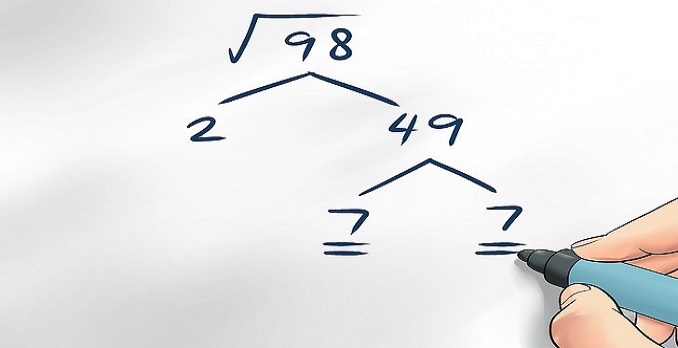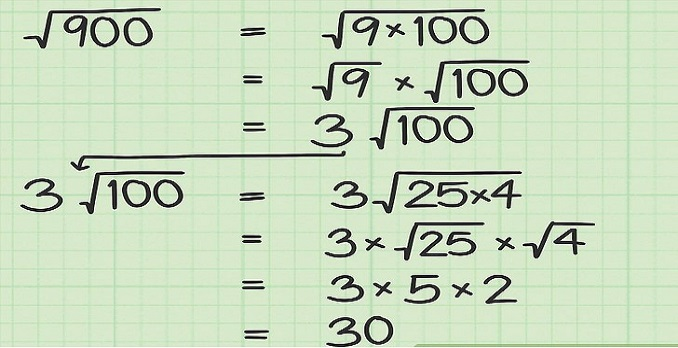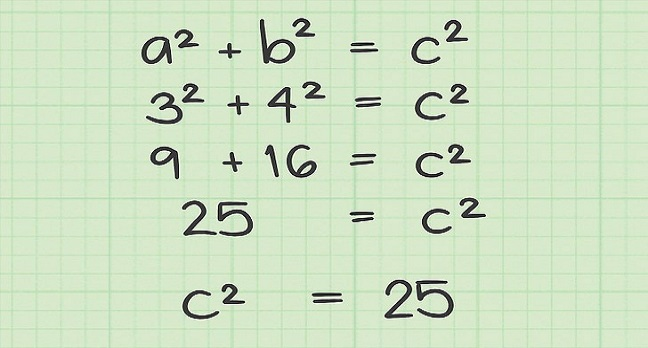# How to calculate a square root without a calculator

The square root is one of the things we all learned at school. Although no one uses it in his professional life, in college, it is necessary to learn to calculate it. Here is the definition of the square root according to Wikipedia: “In mathematics, we call the square root of a number the number which, greater than or equal to zero, when raised to the square, is equal to this first number. We will explain systematically how to calculate a square root with an example.

You may read also be interested: How to learn how to write on a computer

## You will need:

• Paper
• PencilThe best way to explain the square root is to start from a number. Let us take 5836,369. Add a 0 next to 9 to generate pairs of digits.

Let us look for a number which, multiplied by itself, is closest to the first group of numbers on the left, while being smaller (in the example, 58). The result should not exceed 58. Once the number is found, let us put it as a radicand. In our example, the number found is 7 because 7 × 7 = 49.Multiply it by itself. The result (49) is written below the first group of numbers on the left (58). Subtraction must then be made between the two. The result of the subtraction (58-49) is 9. Once the result of the subtraction obtained, let us deduce the following pair of digits (36) after this result. The number obtained therefore corresponds to the union between the result of the previous subtraction and the new deferred figures (ie 936). To continue the extraction of the square root, multiply by 2 the first result (7) and write it just below the previous one.

In this step, we must look for a number x that, coupled to 14 and then multiplied by the new number, results in a number equal to or less than 936. The first digit of the result, which is not zero, Acts of a decimal number, is, generally, the one we seek. The result is added to the number of the root and the number of the auxiliary line. In our example, 93 divided by 14 is roughly equal to 6. The following result of the square root is 6. Add the 6 to the radicand.The result of the previous operation (6 x 146 = 876) falls below the number resulting from the subtraction previously performed (936), and then we subtract these two numbers. Add to the result of this subtraction (60) the group of digits of the following radical (in our example, 36). If the next group is after the decimal point, a decimal point is added to the number of the root. The new number is 6036.

The number of the radicand (76) is multiplied by two (the result is therefore 152). Let us seek a number which, coupled with 152 and then multiplied by itself, gives us a number close to 6036. The operation to be carried out here is 1523 × 3. The result (4569) falls under the previous rest and we perform a new subtraction (the result is 1467). Once the subtraction is done, let us take the next digit group and continue in the same way. Note that the number to be divided on the auxiliary line and the remainder is increasingly higher.

We can do this by trial and error or by dividing the first three digits of the root by the first three digits of the auxiliary line (note that previously it was the first two digits), that is, 603 / 152 (the number we are looking for is 3, since the result is 3.9 and, as we said, the number we need to take is the first).Continue in the same way, multiplying the root by 2 again (ignoring the decimal point). The result of the multiplication is added to the third auxiliary line. Then the first four digits of the remainder (1467) are divided by the result of the multiplication (152), and the root and the number of the auxiliary line (9) are obtained. This number is multiplied by the number of the third auxiliary line and is subtracted from the third remainder. We continue in the same way. If there are no more digits, the square root is complete. In our example, 76.3 is multiplied by 2 after removing the decimal (763 and 763 × 2), which gives us a result of 1526. The result is 14679 (note that it is now the first four digits, whereas they were previously the first three). It is then divided by 1526, which gives us a result of 0.9 (as we said, we take the first number different from zero, even if it is decimal, so the figure we are looking for is 9) . Nine is added to the row of the radicand and the third auxiliary line. We multiply 9 by 15269, which gives us a result of 137421. We subtract this result from 146790, which gives us a result of 9369.

The square root of 5836,369 is 76, 39, with a remainder of 9369. The zero is used only to create a pair. We should note that the previous operation used as an example is not complete. If the operation were continued, the result would be 76.396132 (with six decimal places).